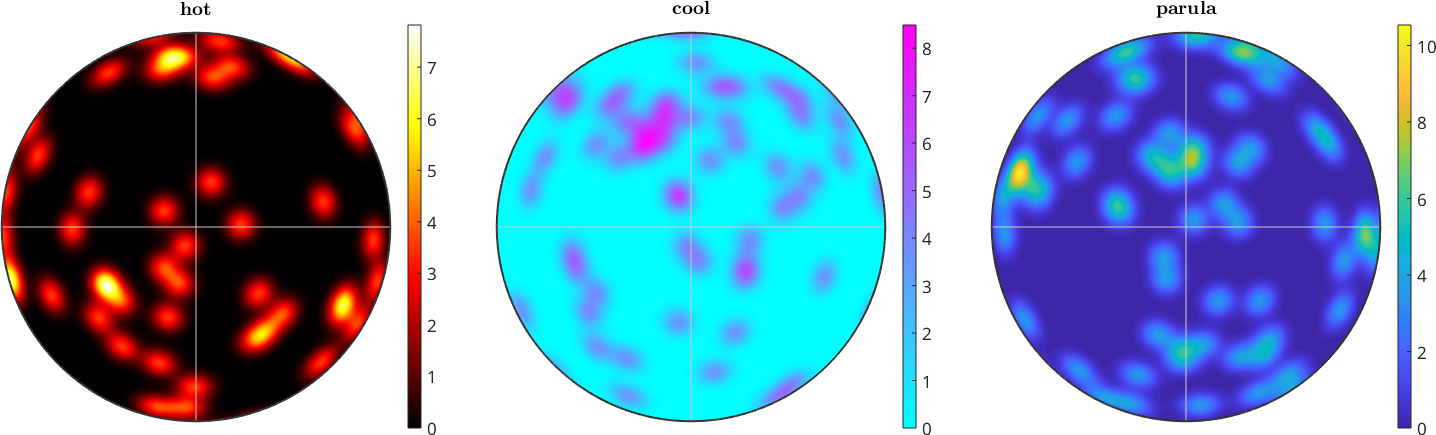Color Mapping edit page

A central issue when interpreting plots is to have a consistent color coding among all plots. In MTEX this can be achieved in two ways. If the the minimum and maximum value are known then one can specify the color range directly using the options colorrange or contourf, or the command setColorRange is used which allows to set the color range afterwards.

## A sample ODFs and Simulated Pole Figure Data

Let us first define some model ODFs to be plotted later on.

## Tight Colorcoding

When plot is called without any colorcoding option, the plots are constructed using the tight option to the range of the data independently from the other plots. This means that different pole figures may have different color coding and in principle cannot be compared to each other.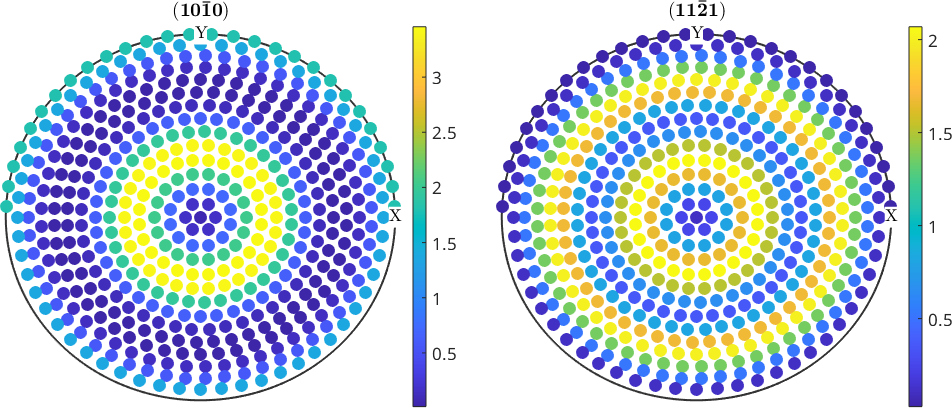## Equal Colorcoding

The tight colorcoding can make the reading and comparison of two pole figures a bit hard. If you want to have one colorcoding for all plots within one figure use the option colorrange to equal.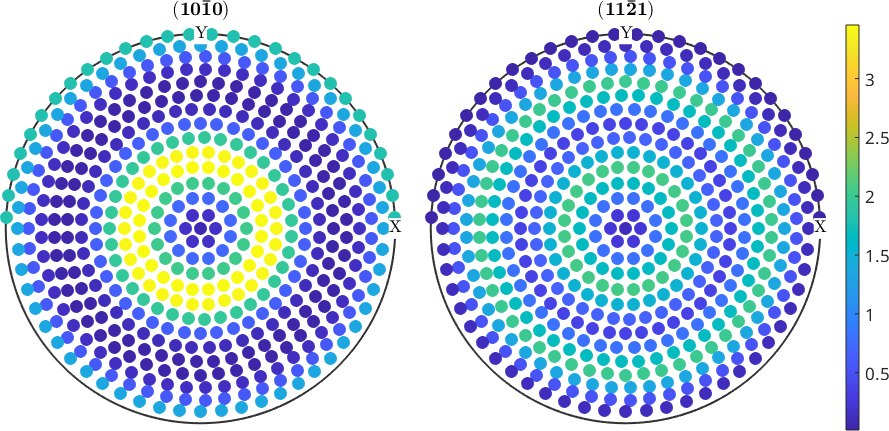## Setting an Explicite Colorrange

If you want to have a unified colorcoding for several figures you can set the colorrange directly in the plot command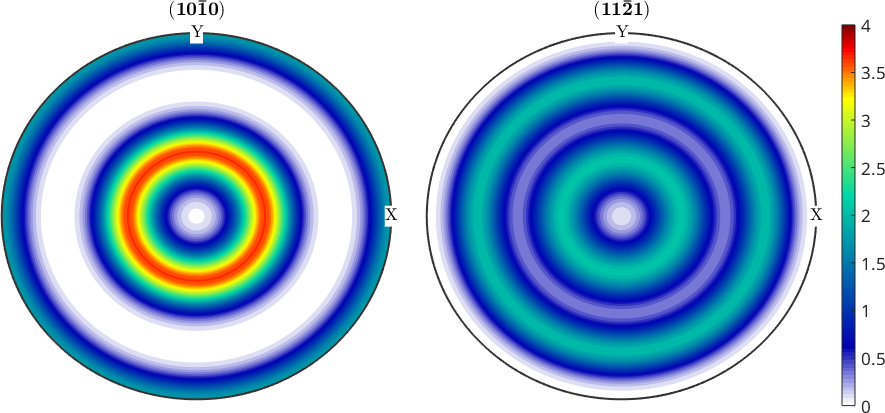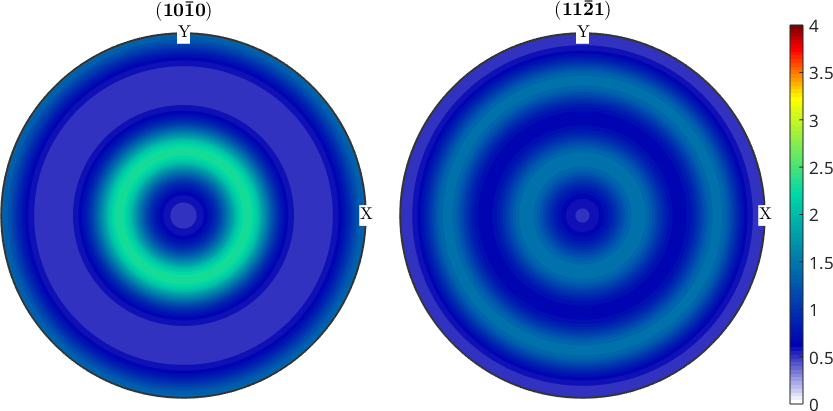## Setting the Contour Levels

In the case of contour plots you can also specify the contour levels directly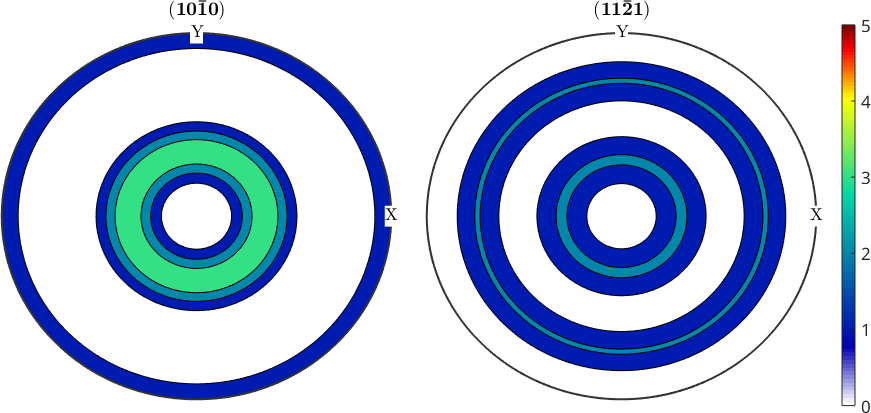## Modifying the Colorrange After Plotting

The color range of the figures can also be adjusted afterwards using the command CLim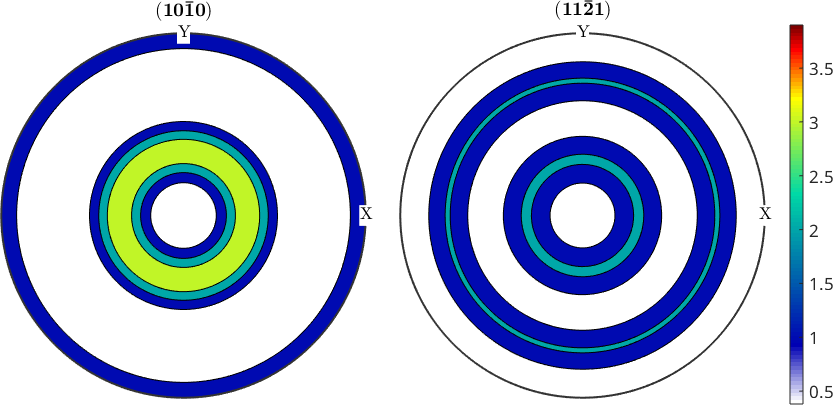## Logarithmic Plots

Sometimes logarithmic scaled plots are of interest. For this case all plots in MTEX understand the option logarithmic, e.g.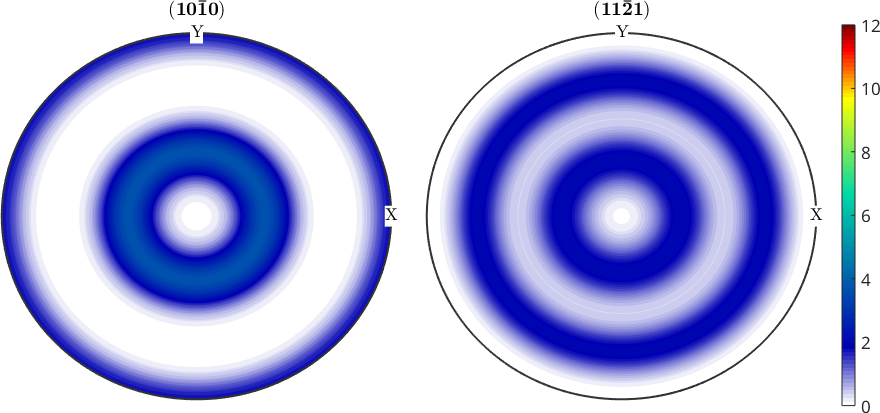## Changing the Colormap

The colormap can be changed by the command mtexColorMap, e.g., in order to set a white to black colormap one has the commands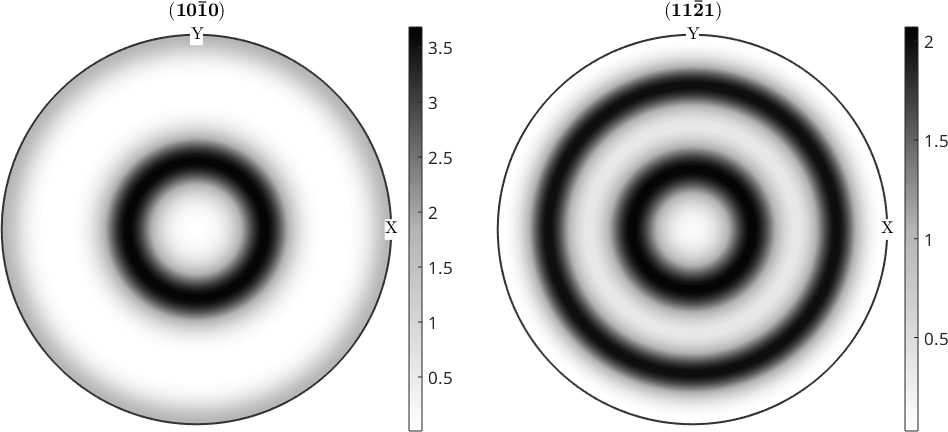## Multiple Colormaps

One can even use different colormaps within one figure